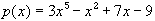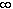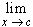#Interactive Real Analysis

Next | Previous | Glossary | Map

## 6.4. Topology and Continuity

### Examples 6.4.9(b):

Show that the equation= 0 has at least one solution in R
Using a computer it is simple enough to draw this function and to see the approximate solution. However, it is even easier to prove that there must be a solution (without specifying where the solution would be).

The function p(x) is an odd-degree polynomial. Therefore:

• If c =, thenp(x) =, so that there exists A such that p(A) > 0
• If c = -, thenp(x) = -, so that there exists B such that p(B) < 0

Hence, by Bolzano's theorem there exists a zero of p(x) between the (unknown !) numbers A and B.

Next | Previous | Glossary | Map